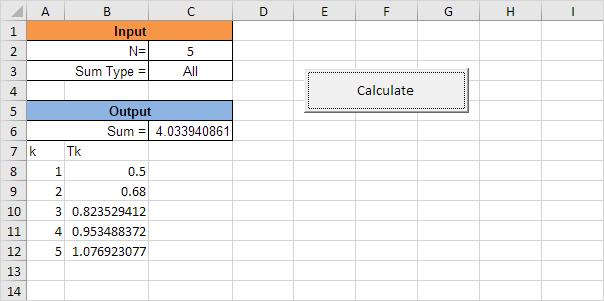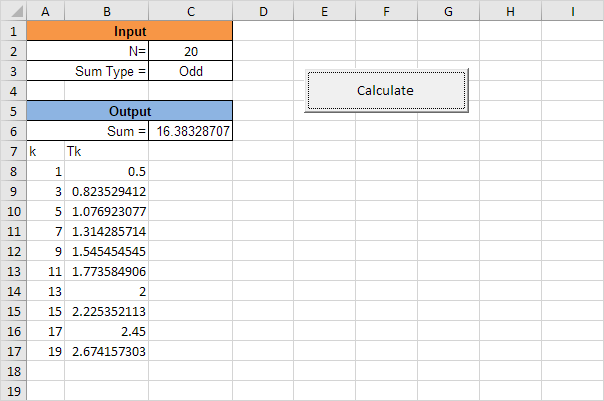# Complex Calculations in Excel VBA

The kth term, Tk, of a certain mathematical series is defined by the following formula:

 Tk = k2 + 6k + 1 9k + 7

The first term, T1, of the series is obtained by substituting k = 1 into the formula i.e.

 T1 = 12 + 6 + 1 = 1 and 9 + 7 2
 T2 = 22 + 12 + 1 = 17 ... and so on 18 + 7 25

Below we will look at a program in Excel VBA that calculates any term Tk and summation of terms up to N.Explanation: the user has the option to enter "All" or "Odd", to respectively calculate the sum of the first N terms of the series or the sum of only the odd terms up to N.

Place a command button on your worksheet and add the following code lines:

1. First, we declare four variables of type Integer and one variable of type String.

Dim i As Integer, term As Integer, N As Integer, stepSize As Integer
Dim sumType As String

2. Second, we initialize the variables.

i = 0
N = Range("C2").Value
sumType = Range("C3").Value

3. Empty the fields.

Range("A8:B1000").Value = ""
Range("C6").Value = ""

4. Determine stepSize.

Select Case sumType
Case Is = "All"
stepSize = 1
Case Is = "Odd"
stepSize = 2
Case Else
MsgBox "Enter a valid expression in cell C3"
End
End Select

Tip: go through our Select Case program to learn more about the Select Case structure.

5. Do the calculations.

For term = 1 To N Step stepSize
Cells(8 + i, 1).Value = term
Cells(8 + i, 2).Value = (term ^ 2 + (6 * term) + 1) / ((9 * term) + 7)

Range("C6").Value = Range("C6").Value + Cells(8 + i, 2).Value

i = i + 1
Next term

Explanation: we use the Step keyword to specify the increment (1 for "All" and 2 for "Odd") for the counter variable of the loop.

Result:Go to Next Chapter: Macro Errors